# Extract Time or Hour Only from a Date and Time in Excel

This post will guide you how to extract time from a data and time value with a formula in Excel. How do I extract time from a date-time number in Excel 2013/2016. How to extract hour only from the date time format in Excel.

## Extract Time from Date and Time

If you want to extract time from a date and time in Excel, you can use a formula based on the TIME function, The HOUR function, the MINUTE function, and the SECOND function. For example, you want to get time value from a date and time value in Cell B1, you can write down the below formula.

`=TIME(HOUR(B1),MINUTE(B1),SECOND(B1))`

#1 Type this formula into a blank cell C1 and press Enter key.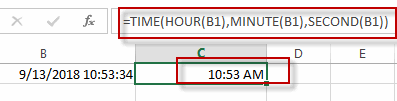#2 Then you need to right click on the Cell C1, and select Format Cell from the pop-up menu list. The Format Cells dialog will open.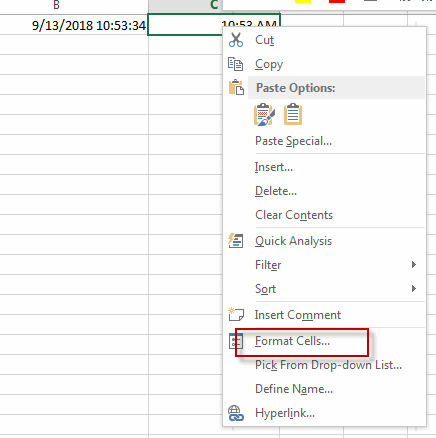#3 switch to Number tab, and click Custom from the Category list. And select one type as: h:mm:ss. Click OK button.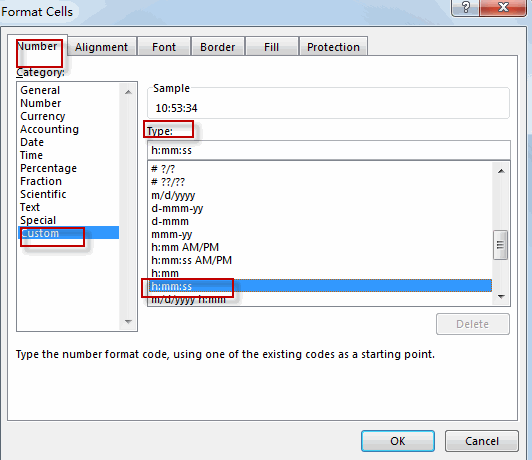#4 let’s see the result.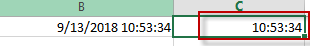## Extract Hour only from Date and Time

If you want to extract hour only, you just need to use the HOUR function. Like this:

`=HOUR(B1)`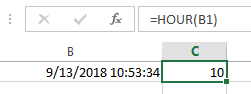If you want to extract minute only from a date and time value, you just need to use the MINUTE function. Like this:

`=MINUTE(B1)`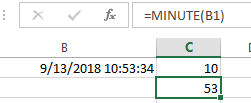If you want to extract second only from a date and time value, you just need to use the SECOND function. Like this:

`=SECOND(B1)`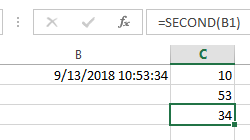### Related Functions

• Excel TIME function
The Excel TIME function returns a date in serial number format for a particular time (the hour, minute and second).The syntax of the TIME function is as below:= TIME(hour, minute, second)…
• Excel HOUR Function
The Excel HOUR function returns the hour of a time value. Or returns an integer value that represent the hour component of a given time. The syntax of the HOUR function is as below:=HOUR (serial_number) …
• Excel MINUTE Function
The Excel MINUTE function returns the minutes of a time value. And the minutes is an integer number from 0 to 59. The syntax of the MINUTE function is as below:=MINUTE (serial_number)…
• Excel SECOND Function
The Excel SECOND function returns the seconds of a time value. Or returns an integer value that represent the second component of a given excel time. And the return value is between 0-59.The syntax of the SECOND function is as below:=SECOND (serial_number)…
Related Posts

VLOOKUP From Another Sheet Not Working

In the previous post, you should know that how to fix or remove the #N/A error when using VLOOKUP formula to lookup value from another sheet. And this post will show you reasons why your VLOOKUP formula is not working ...

If Cell Begins with One of Three Supplied Characters

If you want to test values to see if they begin with some given specific characters like “x”, ”y”, or “z”, you can create a formula with COUNTIF and SUM functions to return results. EXAMPLE You can see “TRUE” or ...

Fix #N/A Error For VLOOKUP From Another Sheet

This post will show you how to fix the #N/A error why it occurs when you extract values from another sheet using VLOOKUP function in Excel 2016,2013,2010 or other Excel versions. How can you correct a #N/A error in VLOOKUP ...

How to Average Only Positive or Negative Numbers of a Range

Suppose both positive numbers and negative numbers exist in a table. If we want to know the average of only positive numbers in this table, we can create a formula to get average of all positive numbers with all negative ...

Sort Positive Numbers and Negative Numbers by Absolute Values

If both positive numbers and negative numbers exist in the same column, when sorting them by absolute values, we can sort them with the help of ABS function and helper column. In this article, we will show you the way ...

Get Employee Information by VLOOKUP

VLOOKUP is one of the key functions among all lookup & reference functions in Excel. Today, in this article, we will show you the way to apply VLOOKUP to retrieve employee information. I hope this article will help you in ...

VLOOKUP with Two Lookup Tables

VLOOKUP is one of the key functions among all lookup & reference functions in Excel. Today we will show you the application of VLOOKUP function when there are two lookup tables. EXAMPLE Table1 and table2 record the rates of Y2020 ...

VLOOKUP with Multiple Lookup Values

VLOOKUP is one of the key functions among all lookup & reference functions in Excel. It can scan and retrieve data from a static or dynamic table based on your lookup value. It can perform approximate match or exact match ...

VLOOKUP Data by Date

VLOOKUP is one of the key functions among all lookup & reference functions in Excel. It can scan and retrieve data from a static or dynamic table based on your lookup value. It can perform approximate match or exact match ...

VLOOKUP – Retrieve Data from Another Workbook

VLOOKUP is one of the key functions among all lookup & reference functions in Excel. It can scan and retrieve data from a static or dynamic table based on your lookup value. It can perform approximate match or exact match ...

Sidebar